# Ray math

Here you will learn about ray math, including what rays are and how to identify them.

Students will first learn about ray math as part of geometry in 4 th grade.

## What is ray math?

A ray is part of a line that has one endpoint and extends on forever in the opposite direction.

They can be shown on their own.

For example,

They can also be shown within a line.

For example,

When two rays share a common endpoint, they form an angle.

For example,

The vertex of the angle, in this case A, is the end point of the rays. The rays that form the angle are ray AX and ray AG.

Other geometric figures, including polygons, contain rays.

For example,

Starting at the endpoint in the shape and extending forever in the opposite direction forms a ray.

A ray is also used when graphing inequalities.

For example,

The ray on the line above represents the number 7 and everything greater. This can be written as x \geq 7.

### What is ray math?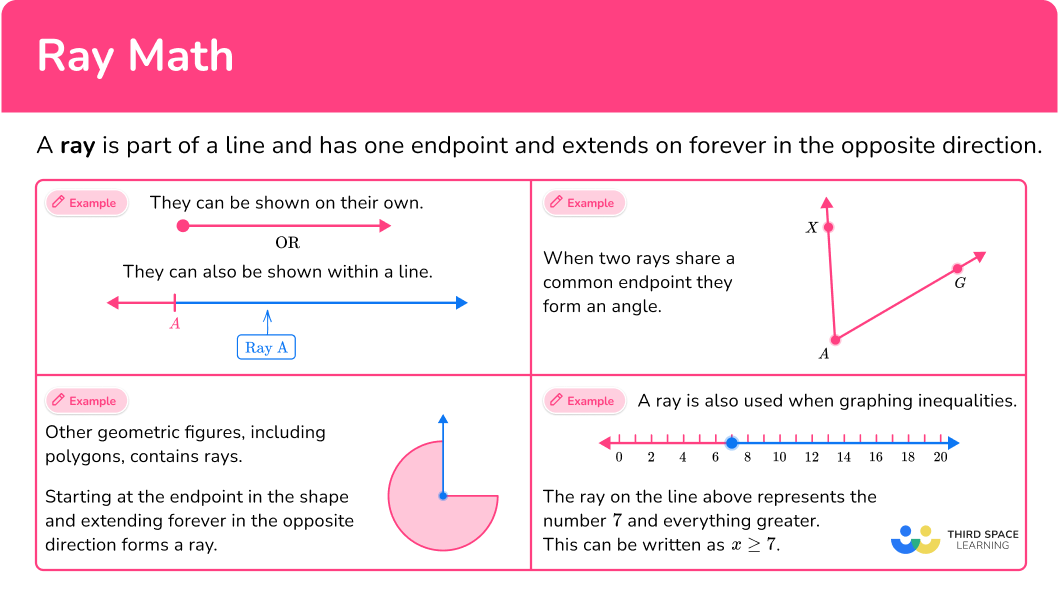## Common Core State Standards

How does this relate to 4 th grade math?

• 4th Grade – Geometry (4.G.A.1)
Draw points, lines, line segments, rays, angles (right, acute, obtuse), and perpendicular and parallel lines. Identify these in two-dimensional figures.

## How to identify rays

In order to identify rays:

1. Look for part of a line.
2. Decide if there is only one endpoint.

## Ray math examples

### Example 1: one dimensional

Is the figure a ray?

1. Look for part of a line.

The figure is a line, with arrows pointing in each direction going on forever.

2Decide if there is only one endpoint.

There are no endpoints shown. The figure is not a ray.

Note: Parts of the line are made up of rays, but these parts are not shown in the figure above.

### Example 2: one dimensional

Name a ray shown.

The complete figure is a line, with arrows pointing in each direction going on forever.

The figure shows a line, with the points G and J. Any of these points can be endpoints of a ray. Let’s look at all the possibilities.

From point G going right forever:

Notice, because the ray includes point J, this can also be called Ray GJ.

From point G going left forever:

From point J going right forever:

From point J going left forever:

Notice, because the ray includes point G, this can also be called Ray JG.

### Example 3: rays in a triangle

How many rays are in the triangle?

The sides of a triangle are made up of parts of lines called line segements.

There are 3 line segments but no rays.

### Example 4: rays in an angle

Name the rays that make up the angle.

There are parts that fall on lines.

Q is an endpoint that connects with R and then goes on forever in the opposite direction. It is ray QR.

Q is an endpoint that connects with K and then goes on forever in the opposite direction. It is ray QK.

### Example 5: rays in a geometric figure

How many rays are in the geometric figure shown below?

There are parts that fall on lines.

There is one endpoint that forms 3 different rays.

### Example 6: rays in the real world

How is a ray of light like a ray in math?

A ray of light is straight, so it is part of a line.

A ray of light has one beginning point – a source of the light. This is like the endpoint of a ray in math.

### Teaching tips for ray math

• Worksheets are a good way to practice identifying rays, especially ones that include practice problems with angles. However, there are also opportunities for students to practice identifying and creating rays outside of worksheets. Look for and take advantage of these types of opportunities.

### Easy mistakes to make

• Confusing lines and rays
Lines go on forever in opposite directions, whereas rays have a fixed starting point.

• Thinking there is not a ray because it is not marked
There are an infinite amount of rays in a line, even if they are not marked.
For example,
The figure below is a line. It is NOT a ray.

However, it has many rays within it. By choosing a point and then continuing forever in the opposite direction, you can name a ray within the line.

### Practice ray questions

1) Which figure shows ray AB?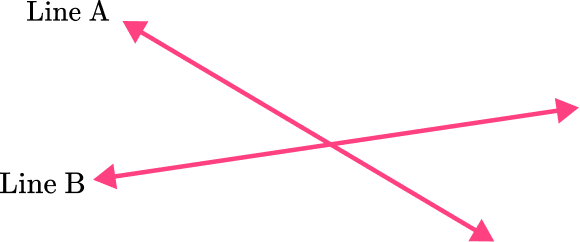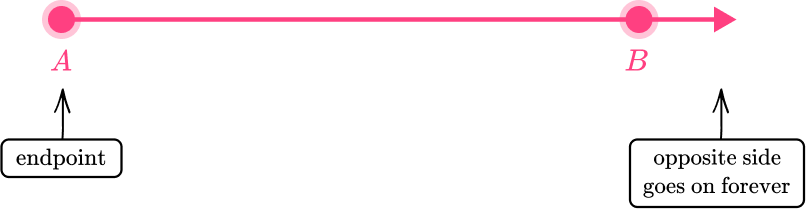Based on the ray definition, this figure is part of a line and has one endpoint.

2) Does connecting points C and G form a ray? Why or why not?Yes, because there are two endpoints.No, because there are two endpoints.Yes, because it is part of a line.No, because it is not part of a line.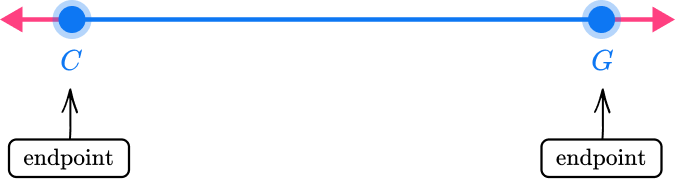This figure has two clear endpoints. A ray only has one endpoint. Connecting points C and G does NOT form a ray.

3) Which shape has exactly 2 rays?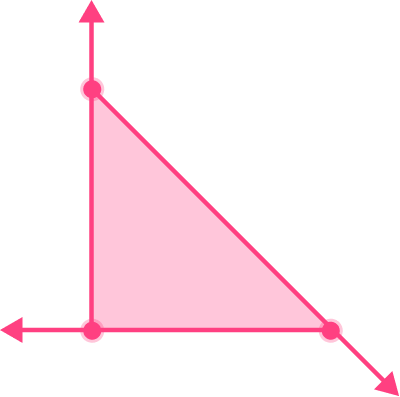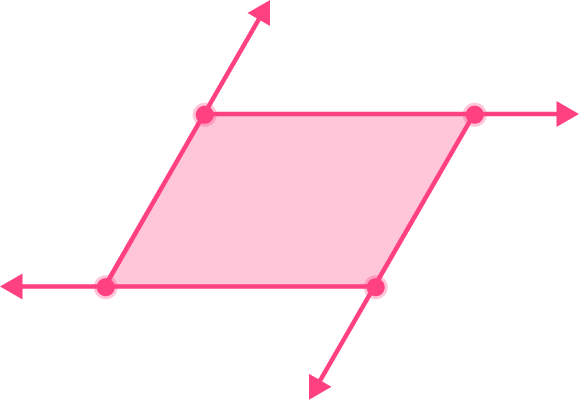The side connected by the points is straight, which means it is part of a line.

Starting from an endpoint and going forever in the opposite direction form a ray.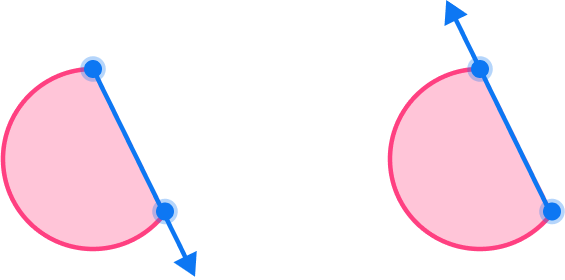This shape has exactly 2 rays.

4) Name the rays that make up the angle.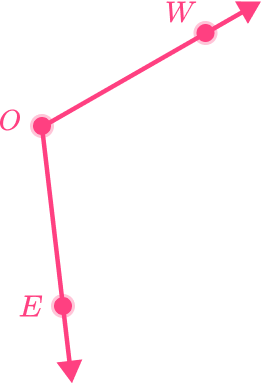ray OW and ray WEray EO and ray OEray OE and ray OWray EW and ray EOO is an endpoint that connects with E and then goes on forever in the opposite direction. It is ray OE.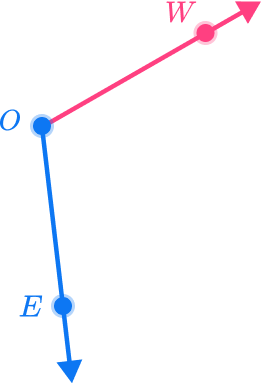O is an endpoint that connects with W and then goes on forever in the opposite direction. It is ray OW.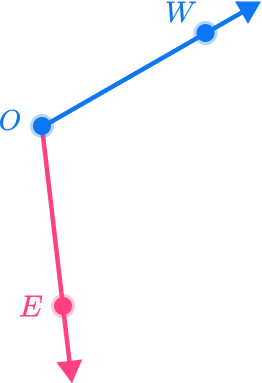5) How many rays are shown in the geometric figure below?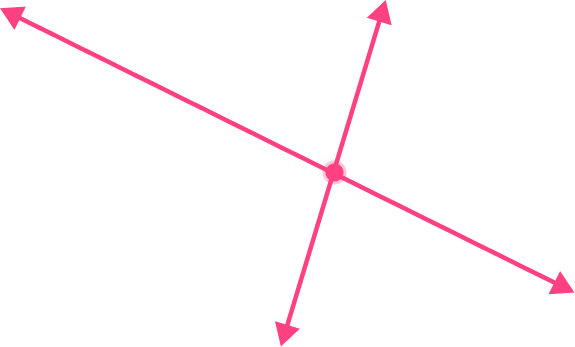4821The point where the lines intersect is an endpoint.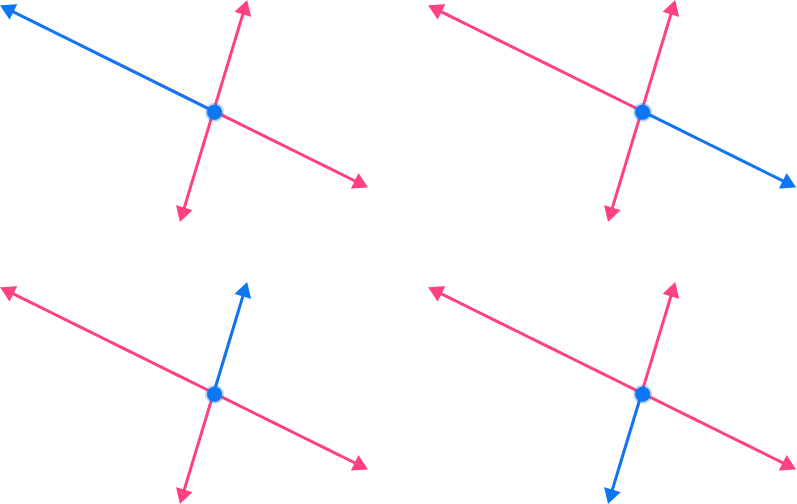6) Which real world shape (shown in blue) best represents a ray?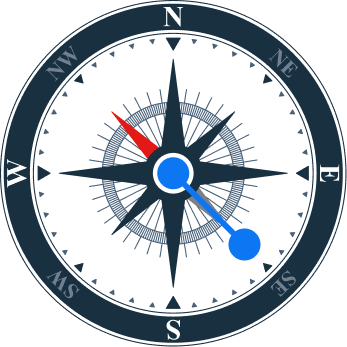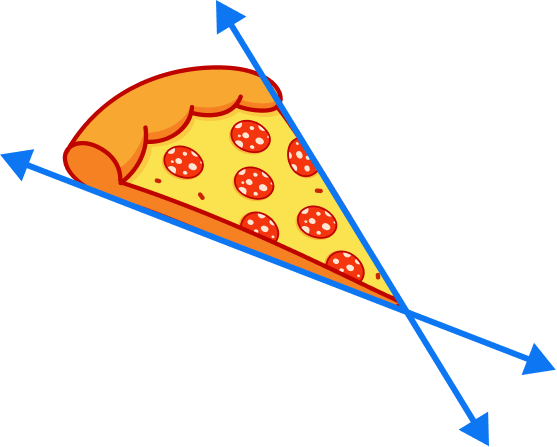The light coming from the sun, sun rays, has a clear starting point and extends forward as part of a line. It represents a ray.

## Ray math FAQs

Are there rays on a number line?

If you choose a starting point (any number – including fractions and decimals) and continue forever in an opposite direction, it will form a ray.

What are opposite rays?

Opposite rays have the same endpoint but extend in opposite directions. Together they form a line.

What are vectors?

A vector is represented like a ray (with an endpoint and an arrow), but the arrow is only used to indicate direction. Vectors and rays are not the same. Vectors are introduced in high school.

What can you do if you need math help?

You can use websites, like this, that overview a topic and provide practice. You can also work with test prep material. If the option is available to you, math tutoring lets you work with someone who understands the topic and can help teach it to you.

## Still stuck?

At Third Space Learning, we specialize in helping teachers and school leaders to provide personalized math support for more of their students through high-quality, online one-on-one math tutoring delivered by subject experts.

Each week, our tutors support thousands of students who are at risk of not meeting their grade-level expectations, and help accelerate their progress and boost their confidence.